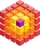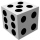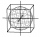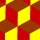# Cube + fractions - math problems

#### Number of problems found: 11

• Sq and cubeFind the product of the square of (1/2) and the cube of (2/3)
• One diceCalculate the probability of one dice roll with the numbers 1, 2, 3, 4, 5, 6 on the walls. Write the results in a notebook in the shape of a fraction in the basic form: 2/3. a, The number 1 falls on the cube. b, The number 5 falls on the cube. c, An even
• Water containerThe cube-shaped container is filled to two-thirds of its height. If we pour 18 liters, it will be filled to three-fifths of the height. What is the volume of the whole container?
• Container with waterThe cube-shaped container is filled with water to half its height. If we add 20 liters of water, the container will be filled to three-quarters of its height. What is the volume of the whole container?
• Dice and coinA number cube is rolled and a coin is tossed. The number cube and the coin are fair. What is the probability that the number rolled is greater than 2 and the coin toss is head?
• Profit growthThe profit of a company increased by 25% during the year 1992, increased by 40% during the year 1993, decreased by 20% in 1994 and increased by 10% during the year 1995. Find the average growth in the profit level over the four years periods?
• The cubeThe cube has an edge of 12 dm. The second cube has an edge exactly 20% longer. How many % is more water in the second cube than in the first cube, if the first cube is full to 3/4 and the second to 3/8?
• SeawaterSeawater has a density of 1025 kg/m3, ice 920 kg/m3. 8 liters of seawater froze and created a cube. Calculate the size of the cube edge.
• Cube, cuboid, and sphereVolumes of a cube and a cuboid are in ratio 3: 2. Volumes of sphere and cuboid are in ratio 1: 3. At what rate are the volumes of cube, cuboid, and sphere?
• Cube in a sphereThe cube is inscribed in a sphere with a volume 7253 cm3. Determine the length of the edges of a cube.
• Cube cutIn the ABCDA'B'C'D'cube, it is guided by the edge of the CC' a plane witch dividing the cube into two perpendicular four-sided and triangular prisms, whose volumes are 3:2. Determine in which ratio the edge AB is divided by this plane.

We apologize, but in this category are not a lot of examples.
Do you have an exciting math question or word problem that you can't solve? Ask a question or post a math problem, and we can try to solve it.

We will send a solution to your e-mail address. Solved examples are also published here. Please enter the e-mail correctly and check whether you don't have a full mailbox.

Need help to calculate sum, simplify or multiply fractions? Try our fraction calculator. Cube Problems. Fraction Word Problems.